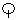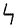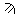﻿ KATERINA SARRI WEBTOPOS - Greek letters as numbers Ancient greek numerals

The ancient greeks used their alphabet letters as numbers. There were various versions of systems through times (see links).

The system still used in some cases (e.g. in very very official documents) is the standard system:
A small or capital letter followed by an acute diacritic mark is the symbol of a number in greek numerical system. If preceded by a stroke under the letter, it is the same number, multiplied by a thousand: e.g.:α´= 1 but ,α= 1000, or = 70 but ,o= 70000

CHECK THE ALPHABET CHART for all numbers, including koppa, sampi and the so-called stigma: Stigma can be written with the two letters sigma+tau στ pronounced [st], or alternatively by the final sigma ς. It represents number 6, with normally would have been the early abandoned 6th letter digamma.

α(1) β(2) γ(3) δ(4) ε(5) στ or ς (stigma is 6) ζ(7) η(8) θ(9)
ι(10) κ(20) λ(30) μ(40) ν(50) ξ(60) ο(70) π(80)or(koppa abandoned letter is 90)
ρ(100) σ(200) τ(300) υ(400) φ(500) χ(600) ψ(700) ω(800)(the sampi 'quasi Pi' is 900)

now, just put them together: e.g. the year ,βζ´ (2007)

Links for ancient greek numbers (systems, fractions, etc.)
Chiffres grecs Greek numbering systems in french both Herodian and classic number symbols
Greek numbers at st-andrews.ac.uk
Greek Numbers and Arithmetic by Prof. Donald Allen USA
Correspondences Between Hebrew, Greek and Roman Alphabets Numerals by John Opsopaus